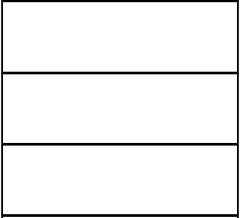# Length and width

Use the 5-step procedure. The perimeter of a rectangle is 34 in. The length is 4 in less than three times the width. Find the length and width.

l =  11.75 in
w =  5.25 in

### Step-by-step explanation:Did you find an error or inaccuracy? Feel free to write us. Thank you!

Tips for related online calculators
Do you have a linear equation or system of equations and looking for its solution? Or do you have a quadratic equation?

#### Grade of the word problem:

We encourage you to watch this tutorial video on this math problem: